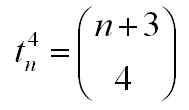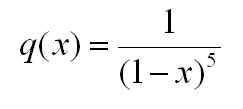## Friday, August 7, 2009

### triangulo-triangular numbersI recently learned from Janet Beery's paper, Formulating Figurate Numbers, that mathematicians have been referring to the 4-dimensional triangular numbers as the 'triangulo-triangular' numbers for about 400 years. Here are the 'triangular' numbers of dimension 0 through 5:These are built by summing (or stacking, if you like the visual analogy) triangular numbers of one dimension less:I've stumbled across the triangulo-triangulars a few times recently - here are two places where you see them.

1. upper triangle sums in multiplication tables

Take a standard school multiplication table and split it along the diagonal that goes from bottom left to upper right. If you sum up all the numbers from the left hand side (including the middle diagonal), you get a triangulo-triangular number.You can work out why this happens using ideas in this post.

2. connecting n+3 points on a circle

If you place n+3 points on a circle and connect them all, then the maximum number of line crossings in the circle is the nth triangulo-triangular number.The triangulo-triangulars come up in this context because to get a crossing you have to choose four of the n+3 points on the circle (drawing lines between the four chosen points gives you your intersection point), and "n+3 choose 4" gives you the triangulo-triangulars. You can see this also in how they occur in the r=4 column of Pascal's Triangle:Just as they show up in several contexts, there are several ways to express a formula for the triangulo-triangulars. The direct formula below shows their quartic nature:and the direct formula comes easily from the combinatorial one:There is also a nice ordinary generating function for them, as described here.